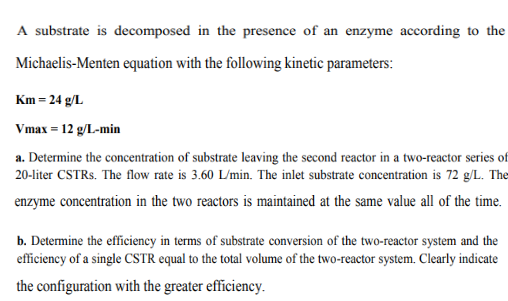# A substrate is decomposed in the presence of an enzyme according to theMichaelis-Menten equation with the following kinetic parameters:Km 24 g/LVmax - 12 g/L-mina. Determine the concentration of substrate leaving the second reactor in a two-reactor series of20-liter CSTRs. The flow rate is 3.60 L/min. The inlet substrate concentration is 72 g/L. Theenzyme concentration in the two reactors is maintained at the same value all of the time.b. Determine the efficiency in terms of substrate conversion of the two-reactor system and theefficiency of a single CSTR equal to the total volume of the two-reactor system. Clearly indicatethe configuration with the greater efficiency.

Question
31 viewshelp_outlineImage TranscriptioncloseA substrate is decomposed in the presence of an enzyme according to the Michaelis-Menten equation with the following kinetic parameters: Km 24 g/L Vmax - 12 g/L-min a. Determine the concentration of substrate leaving the second reactor in a two-reactor series of 20-liter CSTRs. The flow rate is 3.60 L/min. The inlet substrate concentration is 72 g/L. The enzyme concentration in the two reactors is maintained at the same value all of the time. b. Determine the efficiency in terms of substrate conversion of the two-reactor system and the efficiency of a single CSTR equal to the total volume of the two-reactor system. Clearly indicate the configuration with the greater efficiency. fullscreen
check_circle

Step 1

Part (a)

Let the reaction taking place in the CSTR be,

S→P

According to the Michaelis-Menten equation, the reaction rate for this reaction is defined as written below.

Step 2

For two CSTR’s in series;

Volume of each CSTR is, V = 20 L

Volumetric flowrate is, V̇ = 3.60 L/min

Initial concentration of the substrate is, [S0] = 72 g/L

Step 3

Now, calculate the convers...

### Want to see the full answer?

See Solution

#### Want to see this answer and more?

Solutions are written by subject experts who are available 24/7. Questions are typically answered within 1 hour.*

See Solution
*Response times may vary by subject and question.
Tagged in

### Chemical Engineering